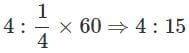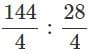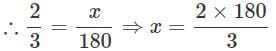# Olympiad Test: Ratio & Proportion

Test Description

## 20 Questions MCQ Test Mathematics (Maths) Class 6 | Olympiad Test: Ratio & Proportion

Olympiad Test: Ratio & Proportion for Class 6 2023 is part of Mathematics (Maths) Class 6 preparation. The Olympiad Test: Ratio & Proportion questions and answers have been prepared according to the Class 6 exam syllabus.The Olympiad Test: Ratio & Proportion MCQs are made for Class 6 2023 Exam. Find important definitions, questions, notes, meanings, examples, exercises, MCQs and online tests for Olympiad Test: Ratio & Proportion below.
Solutions of Olympiad Test: Ratio & Proportion questions in English are available as part of our Mathematics (Maths) Class 6 for Class 6 & Olympiad Test: Ratio & Proportion solutions in Hindi for Mathematics (Maths) Class 6 course. Download more important topics, notes, lectures and mock test series for Class 6 Exam by signing up for free. Attempt Olympiad Test: Ratio & Proportion | 20 questions in 20 minutes | Mock test for Class 6 preparation | Free important questions MCQ to study Mathematics (Maths) Class 6 for Class 6 Exam | Download free PDF with solutions
 1 Crore+ students have signed up on EduRev. Have you?
Olympiad Test: Ratio & Proportion - Question 1

### Identify the ratio of 4 seconds and 1/4 minute from the following.

Detailed Solution for Olympiad Test: Ratio & Proportion - Question 1

Convert 14 minute to seconds and find the ratio.Olympiad Test: Ratio & Proportion - Question 2

### A poster is 1.5 cm long and 7.5 cm wide. Which of the following is the ratio (in lowest terms) of the length and perimeter of the poster?

Detailed Solution for Olympiad Test: Ratio & Proportion - Question 2

Perimeter = 2(L+B)

P = 2(1.5+7.5) = 18

Length:Perimeter = 1.5:18

L:P = 1:12

Olympiad Test: Ratio & Proportion - Question 3

### The length and width of a tape are 2 m and 28 cm. What is their ratio?

Olympiad Test: Ratio & Proportion - Question 4

What is the new ratio obtained by adding 4 to the antecedent and 2 to the consequent of the ratio 3:8?

Detailed Solution for Olympiad Test: Ratio & Proportion - Question 4
• The original ratio was 3:8, which means there are 3 parts of one quantity and 8 parts of another quantity.
• By adding 4 to the antecedent (3 + 4 = 7), we increase the first part of the ratio to 7.
• And by adding 2 to the consequent (8 + 2 = 10), we increase the second part of the ratio to 10.

So, the new ratio would be 7:10.

Olympiad Test: Ratio & Proportion - Question 5

In a cricket coaching camp, 1200 children are trained out of which 900 are selected for various matches. What is the ratio of non-selected children to the total number of children?

Olympiad Test: Ratio & Proportion - Question 6

What is the condition for two ratios to be equal?

Olympiad Test: Ratio & Proportion - Question 7

What are the extremes of the proportion 9:3::36:12?

Detailed Solution for Olympiad Test: Ratio & Proportion - Question 7

Extremes are the end terms. So, 9 and 12 area the extremes of the given proportion.

Olympiad Test: Ratio & Proportion - Question 8

What is the value of x in 12:3: :x:1?

Detailed Solution for Olympiad Test: Ratio & Proportion - Question 8

12:3::x:1  ⇒3x  =12 ⇒ x = 12/3 = 4

Olympiad Test: Ratio & Proportion - Question 9

Which of the following is a proportion?

Detailed Solution for Olympiad Test: Ratio & Proportion - Question 9

In a proportion, product of extremes is equal to product of means.

Olympiad Test: Ratio & Proportion - Question 10

What is the simplest form of the ratio 144:28?

Detailed Solution for Olympiad Test: Ratio & Proportion - Question 10(Since H.C.F. of 144 and 28=4)

Olympiad Test: Ratio & Proportion - Question 11

Find the mean proportion of 25:10::10:4.

Detailed Solution for Olympiad Test: Ratio & Proportion - Question 11

25:10::10:4 102=25 × 4 [ Since b2 = ac ]
∴ 10 is the mean proportion.

Olympiad Test: Ratio & Proportion - Question 12

If x : y : : y : z, identify the correct statement from among the following.

Detailed Solution for Olympiad Test: Ratio & Proportion - Question 12

x:y::y:z ⇒y2=xz

Olympiad Test: Ratio & Proportion - Question 13

Fill in the box so that the three numbers,32 and 64 are in proportion.

Olympiad Test: Ratio & Proportion - Question 14

The ratios 6:3 and 5 :15 are given. Which of the following is true about them?

Olympiad Test: Ratio & Proportion - Question 15

The first, second and the third terms of a proportion are 5,120 and 40. What is the fourth term?

Olympiad Test: Ratio & Proportion - Question 16

The first, third and the fourth terms of a proportion are 6, 12 and 36. What is the second term?

Detailed Solution for Olympiad Test: Ratio & Proportion - Question 16

The ratio of boys and girls = 2:3 Girls =180 Let the number of boys be x=120 ⇒ Boys = 120

Olympiad Test: Ratio & Proportion - Question 17

The ratio of number of boys to number of girls in a tutorial is 2:3. If there are 180 girls, what is the number of boys?

Detailed Solution for Olympiad Test: Ratio & Proportion - Question 17

Given ratio =3:5 lemons = 12 (i.e.,3×4 )water = ? 3 × 4 : 5 × 4 ∴The required number of cups of water =20

Olympiad Test: Ratio & Proportion - Question 18

The ratio of lemons to number of cups of water to be mixed to prepare lemon juice is 3 :5. For 12 lemons what is the number of cups of water required?

Detailed Solution for Olympiad Test: Ratio & Proportion - Question 18

Number of players in a cricket team = 11 Wicketkeeper = 1
∴  Ratio =11:1

Olympiad Test: Ratio & Proportion - Question 19

In a cricket team what is the ratio of total number of players to the number of wicket keepers?

Detailed Solution for Olympiad Test: Ratio & Proportion - Question 19

Total number of players in a cricket team: 11
Number of  Wicket keeper : 1
Ratio 11:1

Olympiad Test: Ratio & Proportion - Question 20

In the word GEOMETRY, what is the ratio of number of consonants to the number of vowels?

## Mathematics (Maths) Class 6

158 videos|282 docs|43 tests(Scan QR code)